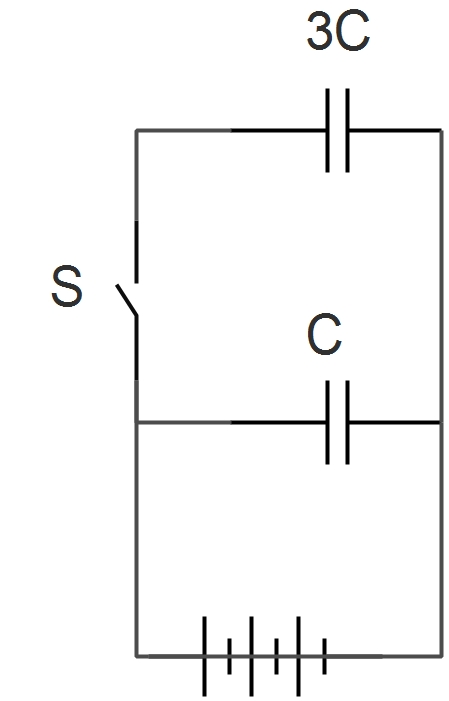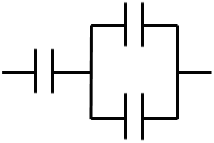Electricity and Magnetism

# Capacitors and Transformers: Level 2-3 ChallengesA transformer has 40 turns in its primary coil and 20 turns in its secondary coil. Is it a step-up transformer or a step-down transformer?

###### Image Credit Wikimedia Commons Brian GreenThe switch in the above figure is closed at $t = 0$. If the capacitor is initially uncharged, then which of the following graphs best represent the current in the circuit at time $t$?Two identical parallel plate capacitors are connected to a battery with the switch $S$ closed. When $S$ is opened and the free space between the capacitors is filled with a material of dielectric constant $K$, the ratio of the total electrostatic energies stored in both capacitors, after and before the introduction of the dielectric, is $\text{\_\_\_\_\_\_\_\_\_\_}$.A parallel plate capacitor of capacitance $10 \ \mu \text{F}$ is charged to $50 \ \mu \text{C}$ using a battery, and is then disconnected from the circuit. How much energy (in microjoules) is required to pull apart the plates such that the distance $d$ between them is doubled?Capacitors in series and parallel

Each capacitor in the above diagram has capacitance $C$. What is the equivalent capacitance?

×